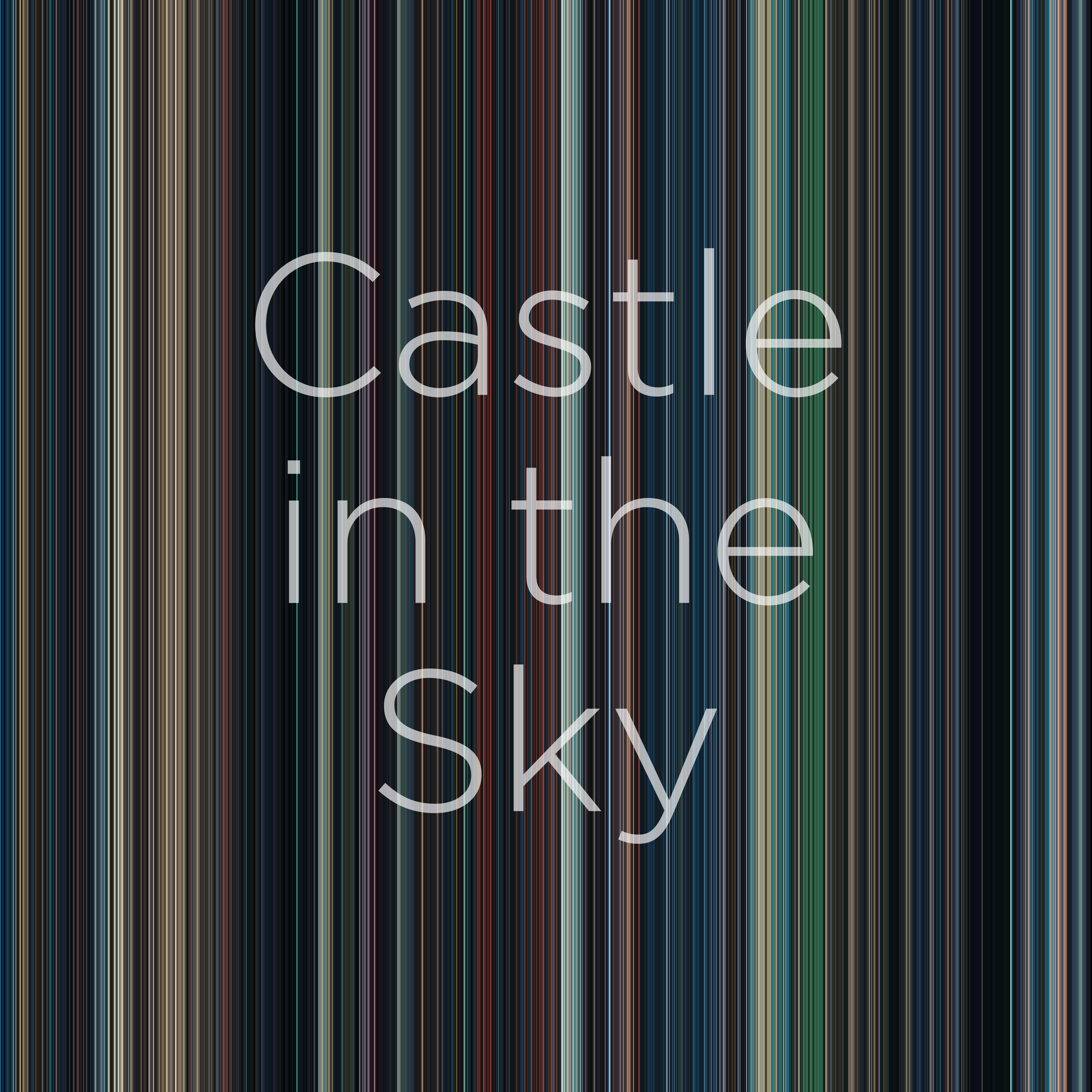# Intro

After coming across some posts online on the clustering of dominant colors in movies presented in a very appealing way, I decided to improve upon my original movies fingerprint algorithm and color palette extractor.

# CodeDev

## Plot arrangement

The first thing I realized whilst looking at other persons’ implementations was that they tended to focus on one color on each of the sampled movie frames, which simplified the presentation of the results. The second thing, that they presented the results in a more appealing way than I originally did.

To remedy the second point, I settled on doing square representations of the colors with a title overlay. In these representation, each vertical bar stands for a sampled frame in the movie:## Improved clustering

Now, on the trickier part, I looked at my code and realized that it worked fine with the case of one cluster for the whole frame. This, however, has one important drawback: as it assigns every pixel to the same cluster and then returns the centroid, so it pretty much calculates the average color of the image (as in the example shown above). This is not a problem for cases in which movies are very skewed in color-space, and is, in fact, the way many of the algorithms found online work; but, I wanted this algorithm to be more general and to be able to detect several clusters even if it only returned a subset. For this extension, a couple of changes were needed: we needed to calculate and detect the required clusters, and then return a subset.

As we can clearly see in the image below, the resulting colors were far brighter, as compared to the original that looked “washed away” due to the centroid being “pulled” from the detected color towards the other colors present in the frame.

def dominantImage(
img, domColNum, clustersNum, maxIter=100
):
(frame, shp) = img
flatFrame = frame.reshape([1, shp * shp, 3])
kMeansCall = MiniBatchKMeans(n_clusters=clustersNum, max_iter=maxIter)
kmeans = kMeansCall.fit(flatFrame)
# Take the color palette and add it to the clusters container
if (domColNum==1 and clustersNum==1):
palette = kmeans.cluster_centers_
clusters[i] = [rescaleColor(color) for color in palette]
else:
frequencies = {
key: len(list(group)) for key, group in groupby(sorted(kmeans.labels_))
}
dominant = dict(
sorted(frequencies.items(), key = itemgetter(1), reverse = True
)[:domColNum])
dominantKeys = list(dominant.keys())
palette = [kmeans.cluster_centers_[j] for j in dominantKeys]
myiter = cycle(palette)
pallettePad = [next(myiter) for _ in range(domColNum)]
colors = [rescaleColor(color) for color in pallettePad]
return colors## Parallelization

For bonus points on the scripts, I decided to parallelize the processing of the frames (as I was a bit tired of waiting for scripts to run). Doing this was a bit trickier than expected, as it required the use of a memmap to be able to modify a common numpy array from different threads.

def parallelDominantImage(
filepaths, domColNum, clustersNum,
maxIter=100, VERBOSE=True, jobs=4
):
mmap = 'memmap.job'
clustersArray = np.memmap(
mmap, dtype=np.double,
shape=(len(filepaths), domColNum, 3), mode='w+'
)
# clustersArray = np.empty((len(filepaths), domColNum, 3))
Parallel(n_jobs=jobs)(
delayed(dominatImageWrapper)(
ix, filepaths, clustersArray,
domColNum, clustersNum,
maxIter=maxIter, VERBOSE=VERBOSE
) for ix in range(0, len(filepaths))
)
os.remove(mmap)
return clustersArray## Bash script

Finally, I coded a bash script to automate the whole process of re-scaling, extracting frames, and exporting the fingerprint.

#!/bin/bash

PT_I=$1 PT_O=$2
FNAME=$3 # "Nausicaa.mp4" TITLE=$4 # "Nausicaä\nof the\nValley\nof the\nWind"
# Constants that shouldn't be constants ---------------------------------------
DOM='5'
CLS='7'
SCALE='640:370' # '480:270'
FRNUM='3600'
DPI='500'
# Internally-generated scratch folders ----------------------------------------
PT_R="$PT_I/Rescaled" PT_F="$PT_I/Frames"
# Create directories ----------------------------------------------------------
mkdir -p $PT_R mkdir -p$PT_F
mkdir -p $PT_O # Rescale movie --------------------------------------------------------------- echo "* Processing:$FNAME"
echo "[1/3] Re-scaling $FNAME..." # ffmpeg -n -loglevel panic -i "$PT_I/$FNAME" -vf "scale=$SCALE" "$PT_R/$FNAME"
echo "[2/3] Exporting frames..."
# python exportFrames.py $FNAME$FRNUM $PT_R$PT_F
echo "[3/3] Generating fingerprint..."
python fingerprint.py "${FNAME%.*}"$DOM $CLS$FRNUM $DPI$PT_F $PT_O "$TITLE"
# Delete scratch folders  -----------------------------------------------------
rm -r $PT_R rm -r$PT_F